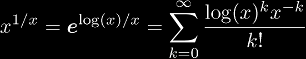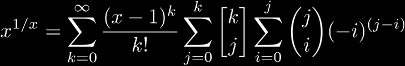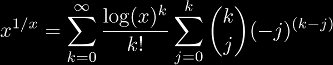# Self-root (x1/x)

The self-root function is a simple function related to tetration. It is the inverse function of the infinite tetrate, and thus it corresponds to the infinite super-root.

# Series for x1/x

Since x1/x is such a simple function, we can expand it directly using the series expansion of the exponential function:The direct function gives the exponential coefficients (1, 1, -2, 3, 4, -90, 786, ...) or (Ax)If we were to substitute x with ex expanding about 0 gives the exponential coefficients (1, 1, -1, -2, 9, -4, -95, 414, ...) or (Ax).